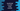# 6 different ways in JavaScript to print the content of an array### 6 different ways in JavaScript to print the content of an array:

In this post, we will learn different ways in JavaScript to print the contents of an array. The arrays are zero-indexed in JavaScript. So, the index of the first item is 0, it is 1 for the second item etc.

We can iterate through each items of the array and print them one by one. By using the index, we can access an element of an array.

Let’s learn how to iterate through the contents of an array by:

• Using a for loop
• Using a while loop
• Using a do-while loop
• Using a forEach loop
• Using a for..of loop
• Using a for..in loop

### Method 1: By using a for loop:

This is the easiest way to iterate through the items of an array. We will use a for loop that will run from 0 to array length - 1. On each iteration, we will access the items of that array by using the current index to print it.

Let’s write the program:

``````let givenArr = ['one', 'two', 'three', 'four'];

for(let i = 0; i< givenArr.length; i++){
console.log(givenArr[i]);
}``````

Here,

• givenArr is the given array. It is an array of strings.
• The for loop runs from i = 0 to i = givenArr.length - 1, i.e. it runs through all of the elements’s indices in the array.
• On each iteration, it increases the value of i by 1.
• Inside the loop, it prints the element it is iterating. The element is accessed by using the index i. The value of i changes on each iteration, so it will print all values of givenArr.

If you run this program, it will print the below output:

``````one
two
three
four``````

### Method 2: By using a while loop:

We can also use a while loop instead of a for loop. The while loop works differently than for loops. The while loop checks for a condition and if the condition is true, it runs the body.

``````let givenArr = ['one', 'two', 'three', 'four'];

let i = 0;

while(i< givenArr.length){
console.log(givenArr[i]);
i++;
}``````

It is almost similar to the for loop example. The only difference is that we are initializing a variable i as 0 before the loop starts.

• The while loop will run until the value of i is less than the length of the array.
• Inside the body of the loop, we are printing the element at index position i.
• After the element is printed, the value of i is incremented by 1. So, in the next iteration, it will print the next element of that array.
• It will stop once the value of i is equal to the length of the array.

If you run this program, it will print the same output.

### Method 3: By using do-while loop:

This is almost similar to the above example. The difference between while and do-while is that do-while loop executes the code before it checks the condition. It runs the code in its body and then it checks the condition. but while loop first checks the condition and then it runs the code in its body.

If we write the above program in do-while, it will look as like below:

``````let givenArr = ['one', 'two', 'three', 'four'];

let i = 0;

do{
console.log(givenArr[i]);
i++;
}while(i< givenArr.length);``````

If you run this program, it will print the contents of givenArr:

``````one
two
three
four``````

But, if the array is empty, it will print undefined.

``````let givenArr = [];

let i = 0;

do{
console.log(givenArr[i]);
i++;
}while(i< givenArr.length);``````

It will print `undefined`. Because, it runs the code before it checks the condition written inside while().

So, if you want to use do-while, you need to add the if block inside the do block.

``````let givenArr = [];

let i = 0;

do{
if(i < givenArr.length){
console.log(givenArr[i]);
}
i++;
}while(i< givenArr.length);``````

### Method 4: By using forEach:

The forEach() method executes a given function for each element of a given array. We can use this method to print the contens of an array as like below:

``````let givenArr = ['one', 'two', 'three', 'four'];

givenArr.forEach((e) => console.log(e));``````

It will print the contents of givenArr.

We can also get the current index in forEach.

``````let givenArr = ['one', 'two', 'three', 'four'];

givenArr.forEach((e,i) => console.log(`givenArr[\${i}] => \${e}`));``````

It will print the index and content of the array as like below:

``````givenArr => one
givenArr => two
givenArr => three
givenArr => four``````

### Method 5: By using for..of:

The for..of loop iterates over an iterable like string, map, array, set etc. We can use this loop to iterate over the contents of a given array:

``````let givenArr = ['one', 'two', 'three', 'four'];

for(let e of givenArr){
console.log(e);
}``````

It will print the items of the array.

### Method 6: By using for..in:

The for..in loop iterates over the properties of an object. It returns the key of each property. So, if we use for..in with an array, we will get the index of each item. Once we get the index, we can access the items in that array.

``````let givenArr = ['one', 'two', 'three', 'four'];

for(let i in givenArr){
console.log(givenArr[i]);
}``````

It will print:

``````one
two
three
four``````